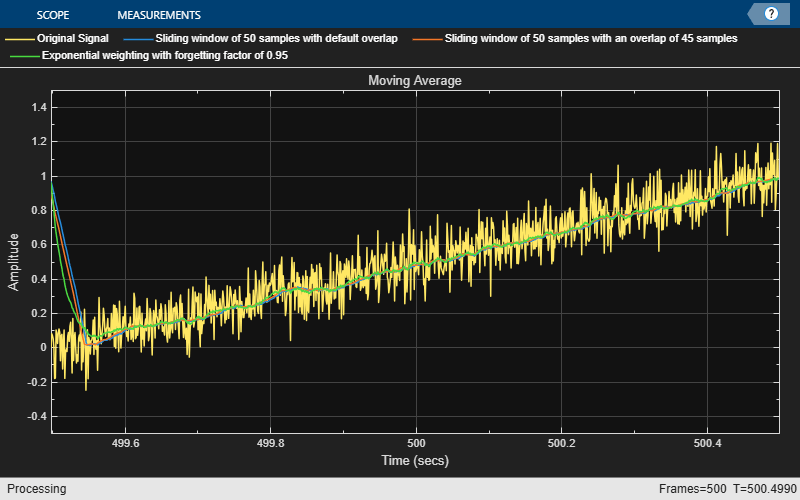# dsp.MovingAverage

Moving average

## Description

The `dsp.MovingAverage` System object™ computes the moving average of the input signal along each channel, independently over time. The object uses either the sliding window method or the exponential weighting method to compute the moving average. In the sliding window method, a window of specified length is moved over the data, sample by sample, and the average is computed over the data in the window. In the exponential weighting method, the object multiplies the data samples with a set of weighting factors. The average is computed by summing the weighted data. For more details on these methods, see Algorithms.

To compute the moving average of the input:

1. Create the `dsp.MovingAverage` object and set its properties.

2. Call the object with arguments, as if it were a function.

## Creation

### Syntax

``movAvg = dsp.MovingAverage``
``movAvg = dsp.MovingAverage(Len)``
``movAvg = dsp.MovingAverage(Name,Value)``

### Description

````movAvg = dsp.MovingAverage` returns a moving average object, `movAvg`, using the default properties.```
````movAvg = dsp.MovingAverage(Len)` sets the `WindowLength` property to `Len`.```

example

````movAvg = dsp.MovingAverage(Name,Value)` specifies additional properties using `Name,Value` pairs. Unspecified properties have default values.Example: ```movAvg = dsp.MovingAverage('Method','Exponential weighting','ForgettingFactor',0.9);``````

## Properties

expand all

Unless otherwise indicated, properties are nontunable, which means you cannot change their values after calling the object. Objects lock when you call them, and the `release` function unlocks them.

If a property is tunable, you can change its value at any time.

Averaging method, specified as `'Sliding window'` or `'Exponential weighting'`.

• `'Sliding window'` — A window of length specified by `SpecifyWindowLength` is moved over the input data along each channel. For every sample the window moves by, the object computes the average over the data in the window.

• `'Exponential weighting'` — The object multiplies the samples with a set of weighting factors. The magnitude of the weighting factors decreases exponentially as the age of the data increases, never reaching zero. To compute the average, the algorithm sums the weighted data.

For more details on these methods, see Algorithms.

Flag to specify a window length, specified as a scalar boolean.

• `true` — The length of the sliding window is equal to the value you specify in the `WindowLength` property.

• `false` — The length of the sliding window is infinite. In this mode, the average is computed using the current sample and all the past samples.

#### Dependencies

This property applies when you set `Method` to ```'Sliding window'```.

Length of the sliding window in samples, specified as a positive scalar integer.

#### Dependencies

This property applies when you set `Method` to `'Sliding window'` and `SpecifyWindowLength` to `true`.

Data Types: `single` | `double` | `int8` | `int16` | `int32` | `int64` | `uint8` | `uint16` | `uint32` | `uint64`

Exponential weighting factor, specified as a positive real scalar in the range (0,1]. A forgetting factor of 0.9 gives more weight to the older data than does a forgetting factor of 0.1. A forgetting factor of 1.0 indicates infinite memory. All the past samples are given an equal weight.

Since this property is tunable, you can change its value even when the object is locked.

Tunable: Yes

#### Dependencies

This property applies when you set `Method` to `'Exponential weighting'`.

Data Types: `single` | `double`

## Usage

### Syntax

``y = movAvg(x)``

### Description

example

````y = movAvg(x)` computes the moving average of the input signal, `x`, using either the sliding window method or exponential weighting method.```

### Input Arguments

expand all

Data input, specified as a vector or a matrix. If `x` is a matrix, each column is treated as an independent channel. The moving average is computed along each channel. The object also accepts variable-size inputs. Once the object is locked, you can change the size of each input channel, but you cannot change the number of channels.

Data Types: `single` | `double`
Complex Number Support: Yes

### Output Arguments

expand all

Moving average of the input signal, returned as a vector or a matrix.

Data Types: `single` | `double`
Complex Number Support: Yes

## Object Functions

To use an object function, specify the System object as the first input argument. For example, to release system resources of a System object named `obj`, use this syntax:

`release(obj)`

expand all

 `step` Run System object algorithm `release` Release resources and allow changes to System object property values and input characteristics `reset` Reset internal states of System object

## Examples

collapse all

Compute the moving average of a noisy ramp signal using the `dsp.MovingAverage` object.

Initialization

Set up `movavgWindow` and `movavgExp` objects. `movavgWindow` uses the sliding window method with a window length of 10. `movavgExp` uses the exponentially weighting method with a forgetting factor of 0.9. Create a time scope for viewing the output.

```FrameLength = 1001; Fs = 1000; movavgWindow = dsp.MovingAverage(10); movavgExp = dsp.MovingAverage('Method','Exponential weighting',... 'ForgettingFactor',0.9); scope = timescope('SampleRate',Fs,... 'TimeSpanOverrunAction','Scroll',... 'ShowGrid',true,... 'YLimits',[-0.5 1.5]); title = 'Sliding Window Average(blue) and Exponentially Weighted Average(red)'; scope.Title = title; ```

Compute the Average

Generate a ramp signal with an amplitude of 1.0 and a time span of 2 seconds. Apply the sliding window average and exponentially weighted average to the ramp. View the output on the time scope.

```for i = 1:500 t = (0:0.001:1)'; unitstep = t>=0; ramp = t.*unitstep; x = ramp + 0.1 * randn(FrameLength,1); y1 = movavgWindow(x); y2 = movavgExp(x); scope([x,y1,y2]); end ```## Algorithms

expand all

 Bodenham, Dean. “Adaptive Filtering and Change Detection for Streaming Data.” PH.D. Thesis. Imperial College, London, 2012.

Watch now